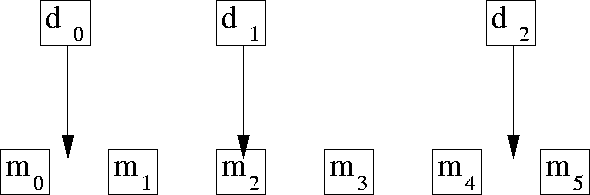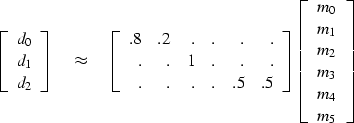Next: Causal integration Up: FAMILIAR OPERATORS Previous: Data-push binning

## Linear interpolation

The linear interpolation operator is much like the binning operator but a little fancier. When we perform the forward operation, we take each data coordinate and see which two model mesh points bracket it. Then we pick up the two bracketing model values and weight each of them in proportion to their nearness to the data coordinate, and add them to get the data value (ordinate). The adjoint operation is adding a data value back into the model vector; using the same two weights, this operation distributes the ordinate value between the two nearest points in the model vector. For example, suppose we have a data point near each end of the model and a third data point exactly in the middle. Then for a model space 6 points long, as shown in Figure 1, we have the operator in (8).

 helgerud Figure 1 Uniformly sampled model space and irregularly sampled data space corresponding to (8).(8)
The two weights in each row sum to unity. If a binning operator were used for the same data and model, the binning operator would contain a 1.'' in each row. In one dimension (as here), data coordinates are often sorted into sequence, so that the matrix is crudely a diagonal matrix like equation (8). If the data coordinates covered the model space uniformly, the adjoint would roughly be the inverse. Otherwise, when data values pile up in some places and gaps remain elsewhere, the adjoint would be far from the inverse.

Subroutine lint1() does linear interpolation and its adjoint.

# Linear interpolation 1-D, uniform model mesh to data coordinates and values.
#
real    f, fx,gx,           o1,d1,coordinate(nd), mm(m1), dd( nd)
do id= 1, nd {
f = (coordinate(id)-o1)/d1;     i=f;     im= 1+i
if( 1<=im && im<m1) {          fx=f-i;   gx= 1.-fx
dd(id) = dd(id) + gx * mm(im) + fx * mm(im+1)
else {
mm(im  ) = mm(im  ) + gx * dd(id)
mm(im+1) = mm(im+1) + fx * dd(id)
}
}
}
return; endNext: Causal integration Up: FAMILIAR OPERATORS Previous: Data-push binning
Stanford Exploration Project
12/26/2000